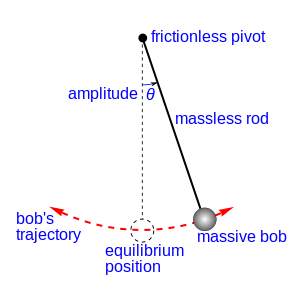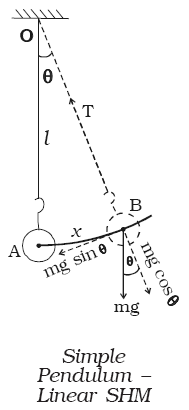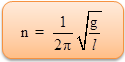Click to Chat

1800-1023-196

+91-120-4616500

CART 0

• 0

MY CART (5)

Use Coupon: CART20 and get 20% off on all online Study Material

ITEM
DETAILS
MRP
DISCOUNT
FINAL PRICE
Total Price: Rs.

There are no items in this cart.
Continue Shopping• Complete Physics Course - Class 11
• OFFERED PRICE: Rs. 2,968
• View Details

Simple Pendulum

Oscillations of a simple pendulumA simple pendulum consists of massless and inelastic thread whose one end is fixed to a rigid support and a small bob of mass m is suspended from the other end of the thread. Let I be the length of the pendulum. When the bob is slightly displaced and released, it oscillates about its equilibrium position. Figure shows the displaced position of the pendulum.

Suppose the thread makes an angle θ with the vertical. The distance of the bob from the equilibrium position A is AB. At B, the weight mg acts vertically downwards. This force is resolved into two components.

(a) The component mg cos θ is balanced by the tension in the thread acting along the length towards the fixed point O.

(b) mg sin θ which is unbalanced, acts A perpendicular to the length of thread. This force tends to restore the bob to the mean position. If the amplitude of oscillation is small, then the path of the bob is a straight line.

∴   F = −mg sin θ            …... (1)

If the angular displacement is small sin θ ≈ θ

F = −mg θ              …... (2)

But  θ = x/l

Thus, F = – mg (x/l)

Comparing this equation with Newton’s second law, F = ma we get,

acceleration a = -gx/l          …... (3)(negative sign indicates that the direction of acceleration is opposite to the displacement) Hence the motion of simple pendulum is SHM.

We know that a = −ω2x

Comparing this with (3)

ω2 = g/l    or   ω = √(g/l)              …... (4)

Time period, T = 2π/ω

So,…... (5)

and frequency,…... (6)

Laws of pendulum

From the expression for the time period of oscilations of a pendulum the following laws are enunciated.

(i) The law of length

The period of a simple pendulum varies directly as the square root of the length of the pendulum.

(i.e) T ∝ √l

(ii) The law of acceleration

(i.e) T ∝ l/√g

The period of a simple pendulum varies inversely as the square root of the acceleration due to gravity.

(iii) The law of mass

The time period of a simple pendulum is independent of the mass and material of the bob.

(iv) The law of amplitudeThe period of a simple pendulum is independent of the amplitude provided the amplitude is small.

Note : The length of a seconds pendulum is 0.99 m whose period is two seconds.

2 = 2π√(l/g)

So, l = 9814/4π2 = 0.99 m

Oscillations of simple pendulum can also be regarded as a case of angular SHM.

Let θ be the angular displacement of the bob B at an instant of time. The bob makes rotation about the horizontal line which is perpendicular to the plane of motion as shown in figure.

Restoring torque about O is  τ = − mg l sin θ

τ = −m g l θ             [∵ θ is small]             …... (1)

Moment of inertia about the axis =m l 2        …... (2)

If the amplitude is small, motion of the bob is angular simple harmonic. Therefore angular acceleration of the system about the axis of rotation is

α = τ/I = – mglθ / ml2

α = (– g/l)θ              …... (3)

We know that, α = – ω2θ                …... (4)

Comparing (3) and (4), we get,

– ω2θ = (– g/l)θ

angular frequency, ω = √g/l

Time period, T = 2π/ω = 2π√l/g              …... (5)

Frequency, n = 1/2π√g/l                     …... (6)

Refer this video to know more about on, “Simple Pendulum”.A simple pendulum is defined as an object that has a small mass, also known as the pendulum bob, which is suspended from a wire or string of negligible mass.

When displaced, a pendulum will oscillate around its equilibrium point due to momentum in balance with the restoring force of gravity.

A hypothetical pendulum consisting of a weight suspended by a weightless string.

A physical pendulum is the generalized case of the simple pendulum. It consists of any rigid body body that oscillates about a pivot point.

For small amplitudes, the period of a physical pendulum only depends on the moment of inertia of the body around the pivot point and the distance from the pivot to the body's center of mass.

The period is still independent of the total mass of the rigid body. However, it is not independent of the mass distribution of the rigid body. A change in shape, size, or mass distribution will change the moment of inertia and thus, the period.

As with springs, there are a number of properties of pendulum motion that you might be tested on, from frequency and period to kinetic and potential energy.

A simple pendulum consists of a weight suspended on a string or wire.

If the pendulum weight or bob is pulled to a relatively small angle from the vertical and let go, it will swing back and forth at a regular period and frequency.

The major factor involved in the equations for calculating the frequency of a pendulum is the length of the rod or wire, provided the initial angle or amplitude of the swing is small.

The mass or weight of the bob is not a factor in the frequency of the simple pendulum, but the acceleration of gravity is in the equation.

Amplitude is the maximum displacement on either side of the mean position, is called amplitude. In the above figure OA and OB measure amplitude.

Vibration is the motion from the mean position to one extreme, then to other extreme and then back to mean position, is called one vibration.

Oscillation is the motion from one extreme to other extreme, is called one oscillation. Thus, one oscillation is half vibration.

A simple pendulum has time period T = 2 s in air. If the whole arrangement is placed in a nonviscous liquid whose density is ½ times the density of the bob. The time period in the liquid will be,

(a) (2/√2) s         (b) 4 s         (c) 2√2 s      (d) 4√2 s

Solution:

We know that,

T =  2π√(l/geff)

geff = (1-1/2) g = g/2

Thus, T ' =√2 T = 2√2 s

From the above observation, we conclude that, option (c) is correct.Question 1

A simple pendulum suspended form the ceiling of a lift has time period T when the lift is at rest. When the lift falls freely, the time period is

(a) infinite

(b) T/g

(c) zero

(d) g/T

Question 2

Phase of SHM describes

(a) displacement only

(b) direction of motion only

(c) both displacement and direction of motion

(d) neither displacement nor direction of motion

Question 3

Natural frequency of simple pendulum depends upon

(a) its mass

(b) its length

(c)square of its length

(d) square root of its length

Question 4

When K.E energy of SHM is maximum its

(a) P.E is zero

(b) acceleration is zero

(c) restoring force is zero

(d) all P.E acceleration and restoring force are zero

Question 5

Mass attached to a spring executes.

(a) vibratory motion

(b) rotatory motion

(c) S.H.M

(d) both (a) and (c)Q.1
Q.2
Q.3
Q.4
Q.5

a

c

d

d

d

Related Resources:-

You might like to simple harmonic motion.

For getting an idea of the type of questions asked, refer the  Previous Year Question Papers.### Course Features

• 101 Video Lectures
• Revision Notes
• Previous Year Papers
• Mind Map
• Study Planner
• NCERT Solutions
• Discussion Forum
• Test paper with Video Solution